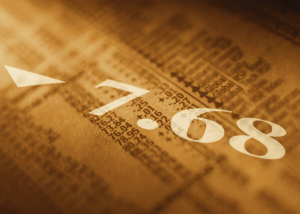# Introduction to statistics

Ronny Gunnarsson. Introduction to statistics [in Science Network TV]. Available at: https://science-network.tv/introduction-to-statistics/. Accessed December 10, 2019.
It is recommended that you read introduction to research before reading this web-page. This web-page gives you a birds perspective of statistics. You will find lots of links from this web-page to other web-pages providing more information.

# Algorithms and mathematics

An algorithm is any set of well defined instructions or steps to accomplish a goal. It could be how to walk from A to B. Mathematical algorithms are precise instructions for how to solve a defined mathematical problem.

Mathematics use numbers in various forms to draw conclusions, usually aiming to solve a problem. It can be purely theoretical problems or practical problems involving obtaining observations from the real world. Mathematical research explores new pathways to make conclusions. Once a pathways to solve a specific problem is established it is usually defined as a mathematical algorithm. Hence, all statistical methods are mathematical algorithms, but not all mathematical algorithms are statistical methods.

# Statistics from a birds perspectiveStatistics is the method used to find a pattern among observations consisting of, or being transformed to, numbers. Hence, statistics is only used in quantitative research approaches and is based on mathematics. The domains embraced by statistics are:

Descriptive statistics try to describe the observations, by using:

Inferential statistics try to condense data and make conclusions from your observations by:

• calculating the probability of being wrong when you reject the null hypothesis described as a the p-value.
• Calculate an effect size such as Cohens d, odds ratios, hazard ratios etc.

# Brief history of statistics

Statistics uses models based on probability theory developed to explain chance variations or random phenomena. The reason it became interesting as a discipline was when mathematicians of the 17th century began calculating the odds in various games of chance. It was obvious that this knowledge has the potential to be financially rewarding. These gaming theories could then be applied into other contexts.

(Under construction)